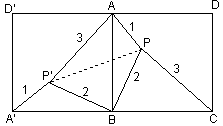# Point in a Square

Inside a square ABCD, a point P is selected such that AP = 1, BP = 2, CP = 3.Find ∠APB.

The following solution is by Murray Klamkin.

Rotate the square through 90° around point B. Let P' be the image of P, A' that of A, and D' of D. Connect P and P'.In ΔBPP', BP = BP' and ∠P'BP is right. The triangle is also isosceles so that ∠BPP' = 45°. From the Pythagorean Theorem, PP'2 = 8.

In ΔAPP', PP'2 + AP2 = AP'2 (8 + 1 = 9). Therefore, by the converse of the Pythagorean Theorem, the triangle is right and ∠P'PA = 90°.

Summing up, ∠APB = ∠BPP' + ∠P'PA = 45° + 90° = 135°.

A similar approach works for a similar problem where point P is at distances 3, 4, 5 to the vertices of an equilateral triangle.

## Reference

1. R. Honsberger, More Mathematical Morsels, MAA, 1991, pp. 4-5.